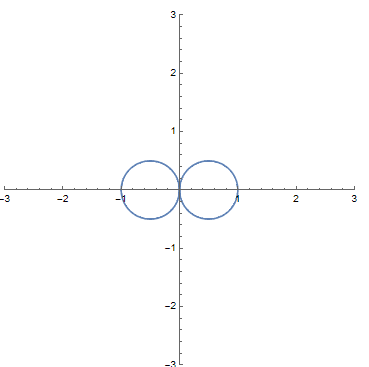# Anti-dual numbers and what are their properties?

• A
• Anixx

#### Anixx

In [this post] user William Ryman asked what would happen if we try to build "complex numbers" with shapes other than circle or hyperbola in the role of a "unit circle".

[Here] I proposed three shapes that could work. The common principle behind them being
that if the unit curve is defined as ##r=r(\phi)##, an arbitrary point, corresponding to a 2-dimensional number on the plane ##z=(a,b)## is characterized by angle ##\alpha(z)=\text{atan2}(b,a)##, magnitude ##M(z)=\frac{\sqrt{a^2+b^2}}{r(\alpha(z))}## and argument ##\operatorname{arg}(z)=\int_0^{\alpha(z)} r(\phi)^2 d\phi##, twice the area of a sector between the radius-vector and ##x## axis.

The addition of numbers is defined element-wise as ##(a_1,b_1)+(a_2,b_2)=(a_1+a_2,b_1+b_2)##.

The multiplication is defined in such a way that the arguments are added and magnitudes are multiplied: ##\operatorname{arg}(uv)=\operatorname{arg}(u)+\operatorname{arg}(v)## and ##M(uv)=M(u)M(v)##.

These definitions make addition and multiplication commutative and associative.

So, I decided to consider the number system based on the following equation for unit curve: ##r=|\cos\phi|##. This function is reciprocal to the function defining dual numbers, so I called the system "anti-dual numbers".The expressions for modulus and argument of a number ##z=(a,b)## thus would be:

##M(z)=\frac{a^2+b^2}{a}##

##\arg z=\frac{1}{2} \left(\frac{a b}{a^2+b^2}+\arctan \left(b/a\right)\right)##

These expressions are valid for the first quarter of the plane, in other quarters we should account that negative modulus corresponds to a shift of argument by ##\pi/2## (not by ##\pi## as in complex numbers!), that's why we have to add the functions arg and mod which are intended to represent the canonical form.

The expression for the angle of direction of radius-vector as a function of argument is [from this post by Tyma Gaidash]:

##\phi (z)=\arcsin\sqrt{I_{\frac{4 \arg z}{\pi }}^{-1}\left(\frac{1}{2},\frac{3}{2}\right)}##

This expression involves [inverse beta regularized] function.

The code below for Mathematica system provides functions for determining argument and modulus of a number ##(a,b)##, determining Cartesian coordinates based on modulus and argument as well as a function that multiplies two numbers given in Cartesian coordinates.

 ar[a_, b_] := 1/2 ((a b)/(a^2 + b^2) + ArcTan[b/a]) m[a_, b_] := (a^2 + b^2)/a arg[a_, b_] := ar[a, b] + Pi/2 Sign[b] HeavisideTheta[-m[a, b]] mod[a_, b_] := Abs[m[a, b]] \[Phi][A_] := ArcSin[Sqrt[InverseBetaRegularized[4 A/Pi, 1/2, 3/2]]] // FullSimplify angle[A_] := Piecewise[{{\[Phi][A], 0 <= A < Pi/4}, {\[Phi][A - Pi/4] + Pi/2, Pi/4 < A <= Pi/2}, {-\[Phi][-A], -Pi/4 < A < 0}, {-\[Phi][-A + Pi/4] - Pi/2, -Pi/2 < A < Pi/4}}] X[m_, A_] := m Cos[angle[A]] Abs[Cos[angle[A]]] Y[m_, A_] := m Sin[angle[A]] Abs[Cos[angle[A]]] Multiply[{a1_, b1_}, {a2_, b2_}] := {X[m[a1, b1] m[a2, b2], ar[a1, b1] + ar[a2, b2]], Y[m[a1, b1] m[a2, b2], ar[a1, b1] + ar[a2, b2]]}

Example:

a := -1; b := -1
arg[a, b]
mod[a, b]

Output:

1/2 (1/2 + Pi/4) - Pi/2
2

Multiplication:

Multiply[{1, 1}, {1, 1}] // N

Output:

{-1.10363, 1.78788}

----------------------

That said, I wonder, what algebraic and analytic properties this system has? It seems to be a 2-dimensional hypercomplex commutative numbering system that is not isomorphic to complex, split-complex and dual numbers.

One interesting feature of this system is existence of divisors of infinity because ##(0,1)(0,1)=\infty## (multiplication by divisors of infinity cannot be handled by the provided code though). This makes the system not closed under multiplication unless an improper element ##\infty## is attached.

What else can be said about the system?

: https://math.stackexchange.com/q/4459901/2513
: https://mathoverflow.net/questions/423657/lemniscate-numbers-and-others-what-would-be-the-properties
: https://i.stack.imgur.com/R3dRX.png
: https://en.wikipedia.org/wiki/Atan2
: https://math.stackexchange.com/a/4390291/2513
: https://mathworld.wolfram.com/RegularizedBetaFunction.html